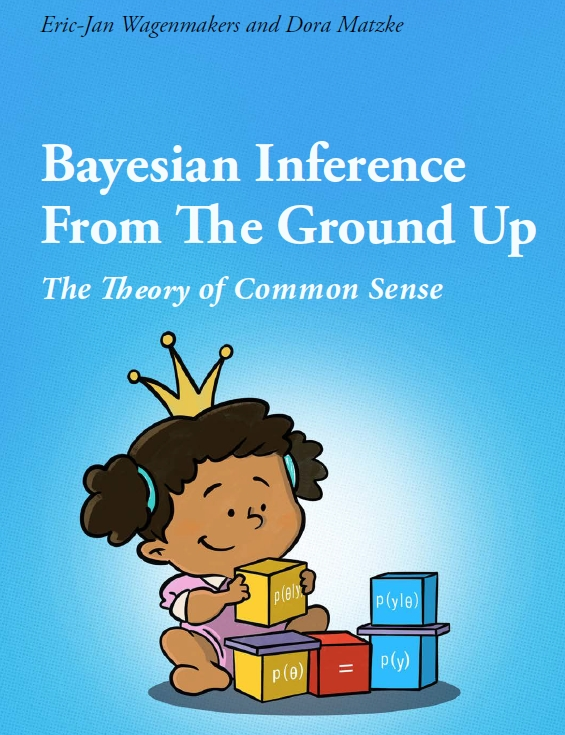# A Free Course Book on Bayesian Inference: [3.] The Rules of Probability

Since 2017, Dora Matzke and I have been teaching the master course “Bayesian Inference for Psychological Science”. Over the years, the syllabus for this course matured into a book (and an accompanying book of answers) titled “Bayesian inference from the ground up: The theory of common sense”. The current plan is to finish the book in the next few months, and share the pdf publicly online. For now, you can click here or on the cover page below to read the first  94 pages. Today we are adding three chapters: “The rules of probability”, “Interlude: Leibniz’s blunder”, and the appendix chapter “Jevons explains permutations”.

Probability is an odd mathematical discipline, as even the greatest scientific minds can struggle with seemingly simple problems. Sometimes, brilliant scholars will analyze a problem in probability theory, arguing that probability is difficult and the results are counterintuitive, provinding elaborate explanations of their proposed solution…and still get it wrong. I am increasingly convinced that the only way to reduce the chance of falling prey to paradoxes in probability is to religiously stick to Bayes’ rule — that is, by manipulating mathematical symbols instead of relying on Venn diagrams or probability trees.References

Wagenmakers, E.-J., & Matzke, D. (in preparation). Bayesian inference from the ground up: The theory of common sense.

Wagenmakers, E.-J., & Matzke, D. (in preparation). Bayesian inference from the ground up: Common sense in practice.